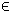## 8LPCON Problem

When solving a problem with a linear objective and nonlinear constraints there is no need to explicitly code the gradient or Hessian. TOMLAB automatically supplies these if lpconAssign is used.

The linear constrained nonlinear programming problem is defined as:

 min x
f(x) = dT x

s/t
 xL ≤ x ≤ xU bL ≤ A x ≤ bU cL ≤ c(x) ≤ cU
(10)
where x, xL, xU, dRn, f(x)R, ARm1 × n, bL,bURm1 and cL,c(x),cURm2.

The following files define an example problem in TOMLAB.

File: tomlab/quickguide/lpconQG.m, lpconQG_c.m, lpconQG_dc.m, lpcon_d2c.m
```  c:   Nonlinear constraint vector
d2c: The second part of the Hessian to the Lagrangian function for the nonlinear constraints.
```
The following file illustrates how to solve this LPCON problem in TOMLAB. Also view the m-files specified above for more information.

File: tomlab/quickguide/lpconQG.m

Open the file for viewing, and execute lpconQG in Matlab.
```% lpconQG is a small example problem for defining and solving linear
% nonlinearly constrained programming problems using the TOMLAB format.

Name = 'Linear constrained problem';
A = [1  0 -1  0
0  1  0 -1
0  0  1 -1];
b_L = [1 1 0]';
b_U = [];
c_L = -1;
c_U = -1;

x_0 = [1 1 1 1]';      % Starting value (not used)

x_L = [-Inf -Inf -Inf 1]'; % Lower bounds for x
x_U = 100*ones(4,1);     % Upper bounds for x

% Objective f = 3*x(1)+2*x(2), assign as linear vector

d = [3 2 0 0]';

Prob = lpconAssign(d, x_L, x_U, Name, x_0, A, b_L, b_U,...
'lpconQG_c', 'lpconQG_dc', 'lpconQG_d2c', [], c_L, c_U);

% Run a global solver for 10000 function evaluations.
Result = tomRun('glcFast', Prob, 1);

% Assign new starting point.
Prob.x_0 = Result.x_k(:,1);

% Run SNOPT as a local solver.

Result2 = tomRun('snopt', Prob, 1);

% Try KNITRO as a local solver, ALG = 3
% Prob.KNITRO.options.ALG = 3;
% Result3 = tomRun('knitro', Prob, 1);```